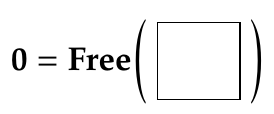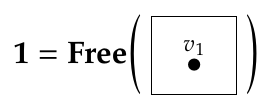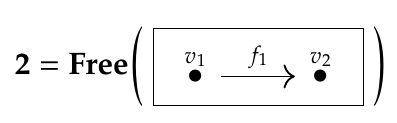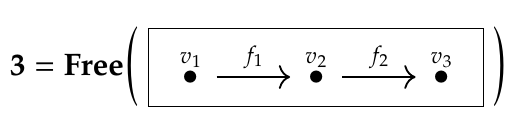> **Puzzle 159.** The categories I've been calling \$$\mathcal{C}\$$ and \$$\mathcal{D}\$$ have other, more purely mathematical names. More precisely, they are _isomorphic_ to two other categories we've already seen in this course, which have more mathematical names. What are those other categories?

Matthew wrote:

> I think \$$\mathcal{C}\$$ has been called "\$$\mathbf{2}\$$" in other discussions and \$$\mathcal{D}\$$ has been called "\$$\mathbf{1}\$$".

That's right! They're part of a sequence of important categories, which are actually posets:and so on.

> Puzzle 159: Is it the arrow category and the terminal category respectively?

That's also write! In previous puzzles we saw that:

* A functor \$$F: \mathbf{1} \to \mathcal{A}\$$ is the same as an object of the category \$$\mathcal{A}\$$.

* A functor \$$F: \mathbf{2} \to \mathcal{A}\$$ is the same as an arrow, or morphism, in the category \$$\mathcal{A}\$$,

* There is exactly one functor \$$F: \mathcal{A} \to \mathbf{1} \$$. Thus, we call \$$\mathbf{1}\$$ the **terminal** category.

* There is exactly one functor \$$F :\mathbf{0} \to \mathcal{A} \$$. Thus, we call \$$\mathbf{0}\$$ the **initial** category.

I didn't introduce the terms 'initial' and 'terminal', but we should!